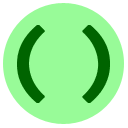# 2X: Extra Practice

This lesson contains extra practice exercises, some of which are challenging. If you get stuck, feel free to skip to the next problem or lesson and come back later. You can always use the My Progress page to review all past exercises.

The first exercise is about debugging. It tries to use a formula that is not quite correct to compute population growth.Coding Exercise: Growth Debugging
Fix the logic error in this program: it should calculate the population of your country for the next 3 years, assuming it starts with 1000 people in 2012, and the number of people increases by 10% each year. You can change at most three characters.

The rest of the exercises in this lesson are about the `min` and `max` functions.

## Simplifying a complex expressionMultiple Choice Exercise: Simplification
What is a simplification of the following expression?

`max(x - 3, min(x + 10, x + 5))`
Correct! Since `x + 5` is always smaller than `x + 10`, we can simplify `min(x + 10, x + 5)` to just `x + 5`. Then working our way outwards, the entire expression is `max(x - 3, x + 5)`. Since `x + 5` is always the maximum of these two numbers, that is the result.

## Complicating a simple expressionCoding Exercise: Complication
Assume that the grader defines two variables `A` and `B` for you. Write a program which prints out the value

`min(A, B)`
However, there is a catch: your program is not allowed to use the `min` function. Instead, use `max` in a clever way to simulate `min`. Hint, Method 1Hint, Method 2

## Payment CalculatorCoding Exercise: Payment Calculator
A credit card company computes a customer's "minimum payment" according to the following rule. The minimum payment is equal to either \$10 or 2.1% of the customer's balance, whichever is greater; but if this exceeds the balance, then the minimum payment is the balance. Write a program to print out the minimum payment using `min` and `max`. Assume that the variable `balance` contains the customer's balance. Your program does not need to print the dollar sign.
Example 1: if your `balance` is `1000`, then your program should print `21`.
Example 2: if your `balance` is `600`, then your program should print `12.6`.
Example 3: if your `balance` is `25`, then your program should print `10`.
Example 4: if your `balance` is `8`, then your program should print `8`.
Hint

## Sorting Scramble

The final problem is a challenging problem, about sorting numbers in a weird way. There are better, simpler, and faster sorting methods that you can learn about after completing our introductory lessons.

If you get stuck, feel free to skip the problem. You can always come back to it later. Additionally, you can keep track of what you have or have not finished by visiting the My Progress page.Scramble Exercise: Sorting Scramble
Code scramble: make the program sort the three numbers `x``y` and `z` into increasing order, so that `x` has the smallest value, `y` has the next smallest value, and `z` has the largest value.
Drag and drop with your mouse to rearrange the lines. Click for a hint.
• tmp = max(x, y)
• y = tmp
• y = min(y, z)
• z = tmp
• tmp = max(x, y)
• x = min(x, y)
• y = tmp
• tmp = max(y, z)
• x = min(x, y)

Continue on to lesson 3!